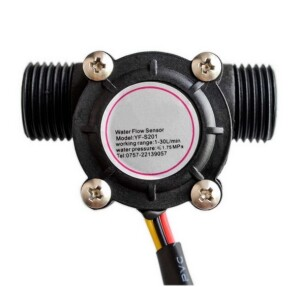Reducere!

## Senzor de debit de apă (Water Flow Sensor) compatibil Arduino

80,00 RON. 49,00 RON.

Senzor umiditate sol (Soil Hygrometer Humidity) pentru proiectele Arduino.

Categorie: Etichete: , , , , , , ,

## Senzor de debit de apă (Water Flow Sensor) compatibil Arduino

Senzor de debit de apă (Water Flow Sensor) compatibil Arduino

YF-S201 1/2”Utilizat în principal la instalatiile pentru testarea apei, sistemul de răcire/incălzire a apei

Aplicație:
încălzitoare de apă
mașini pentru imbuteliat
distribuitor automat de apă
dispozitiv de măsurare a debitului

Amplitudine mare: ≥ 4,6V
Amplitudine redusă: ≤ 0,5V
Testarea presiunii hidrostatice: ≤ 2,0 MPa
Rezistența izolației: ≥ 100MΩ
Temperatura de utilizare: ≤ 80 ° C
Cea mai mică tensiune nominală de lucru: DC4,5 … 5V
Curent maxim de funcționare: 15mA (DC 5V)
Capacitate de încărcare: ≤ 10mA (DC 5V)
Gama de umiditate de funcționare: 35% ~ 90% RH (fără îngheț)
Temperatura: -25 ~ +80 ° C
Racorduri externe: 1/2 ”
2.5 „x 1,4″ x 1,4 ”

5V VCC (red wire)
GND (black wire)
Signal/pulse (usually yellow)

Exemplu cod:

/*
Liquid flow rate sensor

Measure the liquid/water flow rate using this code.
Connect Vcc and Gnd of sensor to arduino, and the
signal line to arduino digital pin 2.

*/

byte statusLed = 13;

byte sensorInterrupt = 0; // 0 = digital pin 2
byte sensorPin = 2;

// The hall-effect flow sensor outputs approximately 4.5 pulses per second per
// litre/minute of flow.
float calibrationFactor = 4.5;

volatile byte pulseCount;

float flowRate;
unsigned int flowMilliLitres;
unsigned long totalMilliLitres;

unsigned long oldTime;

void setup()
{

// Initialize a serial connection for reporting values to the host
Serial.begin(38400);

// Set up the status LED line as an output
pinMode(statusLed, OUTPUT);
digitalWrite(statusLed, HIGH); // We have an active-low LED attached

pinMode(sensorPin, INPUT);
digitalWrite(sensorPin, HIGH);

pulseCount = 0;
flowRate = 0.0;
flowMilliLitres = 0;
totalMilliLitres = 0;
oldTime = 0;

// The Hall-effect sensor is connected to pin 2 which uses interrupt 0.
// Configured to trigger on a FALLING state change (transition from HIGH
// state to LOW state)
attachInterrupt(sensorInterrupt, pulseCounter, FALLING);
}

/**
* Main program loop
*/
void loop()
{

if((millis() – oldTime) > 1000) // Only process counters once per second
{
// Disable the interrupt while calculating flow rate and sending the value to
// the host
detachInterrupt(sensorInterrupt);

// Because this loop may not complete in exactly 1 second intervals we calculate
// the number of milliseconds that have passed since the last execution and use
// that to scale the output. We also apply the calibrationFactor to scale the output
// based on the number of pulses per second per units of measure (litres/minute in
// this case) coming from the sensor.
flowRate = ((1000.0 / (millis() – oldTime)) * pulseCount) / calibrationFactor;

// Note the time this processing pass was executed. Note that because we’ve
// disabled interrupts the millis() function won’t actually be incrementing right
// at this point, but it will still return the value it was set to just before
// interrupts went away.
oldTime = millis();

// Divide the flow rate in litres/minute by 60 to determine how many litres have
// passed through the sensor in this 1 second interval, then multiply by 1000 to
// convert to millilitres.
flowMilliLitres = (flowRate / 60) * 1000;

// Add the millilitres passed in this second to the cumulative total
totalMilliLitres += flowMilliLitres;

unsigned int frac;

// Print the flow rate for this second in litres / minute
Serial.print(„Flow rate: „);
Serial.print(int(flowRate)); // Print the integer part of the variable
Serial.print(„.”); // Print the decimal point
// Determine the fractional part. The 10 multiplier gives us 1 decimal place.
frac = (flowRate – int(flowRate)) * 10;
Serial.print(frac, DEC) ; // Print the fractional part of the variable
Serial.print(„L/min”);
// Print the number of litres flowed in this second
Serial.print(” Current Liquid Flowing: „); // Output separator
Serial.print(flowMilliLitres);
Serial.print(„mL/Sec”);

// Print the cumulative total of litres flowed since starting
Serial.print(” Output Liquid Quantity: „); // Output separator
Serial.print(totalMilliLitres);
Serial.println(„mL”);

// Reset the pulse counter so we can start incrementing again
pulseCount = 0;

// Enable the interrupt again now that we’ve finished sending output
attachInterrupt(sensorInterrupt, pulseCounter, FALLING);
}
}

/*
Insterrupt Service Routine
*/
void pulseCounter()
{
// Increment the pulse counter
pulseCount++;
}

Arduino projects.

## Opinii

Nu există încă recenzii.